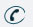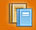781-366-0662

Subject

## Major Areas of Study

Number Sense and Operations : Students will understand number, ways of representing numbers, relationships among number, and number systems. They will understand the meanings of operations and how they relate to one another, compute fluently and make reasonable estimates.

Topics Covered : Order of Operations, Operations with Fractions, Absolute Value, Prime Factorization, Greatest Common Factors, Multiplication and Division Properties of Exponents, Simplifying and Multiplying Rational Expressions

Patterns, Relations, and Algebra : Students will understand patterns, relations, and functions. They will represent and analyze mathematical situations and structures using algebraic symbols, use mathematical models to represent and understand quantitative relationships, and analyze change in various contexts.

Topics Covered : Solving Equations, Function Notation, Composite Functions, Linear Equations and Slope, Parallel and Perpendicular Lines, Linear Inequalities, Matrices, Networks, Problem Solving Via: Substitutions, Elimination and Graphing, Solving 3-Variable Systems, Quadratic Functions, Solving Quadratic Equations Using: Square Roots, The Quadratic Equations, and Factoring, Synthetic Division
(Extensions for levels 1 and/or 2 include graphing functions, domain and range, addition, subtraction, and multiplication of matrices, linear programming, creating non-linear models using power functions, factoring polynomials using long and synthetic polynomial division, creating models using exponential and logarithmic equations, simplifying exponential and logarithmic expressions using properties of logarithms, ‘e’, complex rational functions.)

Geometry : Students will analyze characteristics and properties of two-and three-dimensional geometric shapes and develop mathematical arguments about geometric relationships. They will specify locations and describe spatial relationships using coordinate geometry and other representational systems, analyze transformation and use symmetry to analyze mathematical situations. In addition, they will use visualization, spatial reasoning and geometric modeling to solve problems.

Topics Covered : Geometric Transformations, Pythagorean Theorem, Right Triangle Trigonometry (Extensions for levels 1 and/or 2 include conic sections: foci, radius, axes, directrix, and x/y translations.)

Data Analysis, Statistics, and Probability : Students will formulate questions that can be addressed with data and collect, organize and displace relevant data to answer them. They will select and use appropriate statistical methods to analyze data, develop and evaluate inferences and predictions that are based on data, and understand and apply basic concepts of probability.

Topics Covered : Multiplication Counting Principal, Permutations, Combinations
(Extensions for levels 1and/or 2 include binomial theorem, polynomial expansion and probability, independent and dependent probabilistic events, probabilities of specific sequences, probability distributions, sample bias and sampling error.)Subject Classes